Subject: Irrational numbers Date: Wed, 20 Jan 1999 22:52:55 -0500 If you could help me out with this I would appreciate it. Prove that the square root of 17 is irrational. Thank you for your help. John Hi John Here is a proof that the root of 2 is irrational, taken from the notes of a course I taught last semester. We use what is called a proof by contradiction. The essential idea is that we make an assumption and if we follow that up with a number of logical steps and end up with a false statement, it must be that our assumption was false.    Assume that is rational, in particular assume that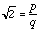where p and q are relatively prime integers. (p and q relatively prime means that they have no common factor except 1, hence p/q cannot be simplified.) Thus,and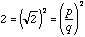. Equivalently,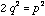. Here's where we use our number theory sense (on divisibility properties)-- we know 2 divides the left side of the equation above hence 2 must divide the right side. But this means that the right side must be even. The only way this can happen is that p itself is even. For simplicity's sake let's write p = 2m. Then we have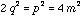and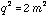But now 2 divides the right side of the equation above and hence 2 divides the left side. The only way this can happen is that q is even also! But then we have both p and q being even when we've already assumed that they were relatively prime. This can't be. Thus, something has gone wrong -- it is our assumption thatwhere p and q are relatively prime integers, i.e. that cannot be rational and thus must be irrational.    Now repeat this argument replacing 2 by 17. The key here is that 2 and 17 are both primes so you need to watch for the place in this argument where it is crutial that 17 is prime. Cheers Harley Go to Math Central To return to the previous page use your browser's back button.